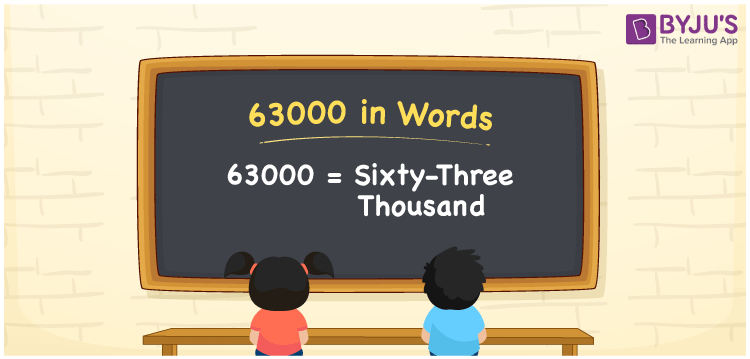# 63000 in words

63000 in words is written as Sixty Three Thousand. 63000 represents the count or value. The article on Counting Numbers can give you an idea about count or counting. The number 63000 is used in expressions that relate to money, distance, length and many more. Let us consider an example of a Stadium crowd capacity. “In a Football stadium with capacity of Sixty Three Thousand, all the 63000 seats were occupied and many were disappointed when the tickets were not available”.

 63000 in words Sixty Three Thousand Sixty Three thousand in Numbers 63000

## 63000 in English Words## How to Write 63000 in Words?

We can convert 63000 to words using a place value chart. The number 63000 has 5 digits, so let’s make a chart that shows the place value up to 5 digits.

 Ten thousand Thousands Hundreds Tens Ones 6 3 0 0 0

Thus, we can write the expanded form as:

6 × Ten thousand + 3 × Thousand + 0 × Hundred + 0 × Ten + 0 × One

= 6 × 10000 + 3 × 1000 + 0 × 100 + 0 × 10 + 0 × 1

= 63000

= Sixty Three Thousand

63000 is the natural number that is succeeded by 62999 and preceded by 63001.

63000 in words – Sixty Three Thousand.

Is 63000 an odd number? – No.

Is 63000 an even number? – Yes.

Is 63000 a perfect square number? – No.

Is 63000 a perfect cube number? – No.

Is 63000 a prime number? – No.

Is 63000 a composite number? – Yes.

## Solved Example

1. Write the number 63000 in expanded form

Solution: 6 x 10000 + 3 x 1000 + 0 x 100 + 0 x 10 + 0 x 1

Or Just 6 x 10000 + 3 x 1000

We can write 63000 = 60000 + 3000 + 0 + 0 + 0

= 6 x 10000 + 3 x 1000 + 0 x 100 + 0 x 10 + 0 x 1

## Frequently Asked Questions on 63000 in words

Q1

### How to write 63000 in words?

63000 in words is written as Sixty Three Thousand.
Q2

### State True or False. 63000 is divisible by 4?

True. 63000 is divisible by 4.
Q3

### Is 63000 divisible by 10?

Yes. 63000 is divisible by 10. It is also divisible by 2, 3, 4, 5, 6, 10, 100 and 1000.